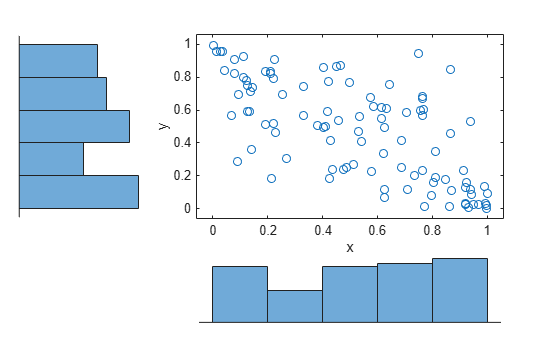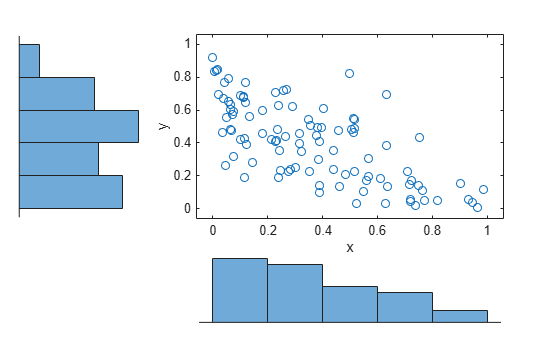copulaparam

Copula parameters as function of rank correlation

Description

example

rho = copulaparam('Gaussian',r) returns the linear correlation parameters, rho, that correspond to a Gaussian copula with Kendall’s rank correlation, r.

rho = copulaparam('t',r,nu) returns the linear correlation parameters, rho, that correspond to a t copula with Kendall’s rank correlation, r, and degrees of freedom, nu.

alpha = copulaparam(family,r) returns the copula parameter, alpha, that corresponds to a bivariate Archimedean copula of the type specified by family, with Kendall’s rank correlation, r.

___ = copulaparam(___,Name,Value) returns the correlation parameter using any of the previous syntaxes, with additional options specified by one or more Name,Value pair arguments. For example, you can specify whether the input rank correlation value is Spearman’s rho or Kendall’s tau.

Examples

collapse all

Generate correlated random data from a beta distribution using a bivariate Gaussian copula with Kendall's tau rank correlation equal to -0.5.

Compute the linear correlation parameter from the rank correlation value.

rng default  % For reproducibility
tau = -0.5;
rho = copulaparam('Gaussian',tau)
rho = -0.7071

Use a Gaussian copula to generate a two-column matrix of dependent random values.

u = copularnd('gaussian',rho,100);

Each column contains 100 random values between 0 and 1, inclusive, sampled from a continuous uniform distribution.

Create a scatterhist plot to visualize the random numbers generated using the copula.

figure
scatterhist(u(:,1),u(:,2))The histograms show that the data in each column of the copula has a marginal uniform distribution. The scatterplot shows that the data in the two columns is negatively correlated.

Use the inverse cdf function betainv to transform each column of the uniform marginal distributions into random numbers from a beta distribution. In the first column, the first shape parameter A is equal to 1, and a second shape parameter B is equal to 2. In the second column, the first shape parameter A is equal to 1.5, and a second shape parameter B is equal to 2.

b = [betainv(u(:,1),1,2), betainv(u(:,2),1.5,2)];

Create a scatterhist plot to visualize the correlated beta distribution data.

figure
scatterhist(b(:,1),b(:,2))The histograms show the marginal beta distributions for each variable. The scatterplot shows the negative correlation.

Verify that the sample has a rank correlation approximately equal to the initial value for Kendall's tau.

tau_sample = corr(b,'type','kendall')
tau_sample = 2×2

1.0000   -0.5135
-0.5135    1.0000

The sample rank correlation of -0.5135 is approximately equal to the -0.5 initial value for tau.

Input Arguments

collapse all

Copula rank correlation, returned as a scalar value or matrix of scalar values.

• If r is a scalar correlation coefficient, then rho is a scalar correlation coefficient corresponding to a bivariate copula.

• If r is a p-by-p correlation matrix, then rho is a p-by-p correlation matrix.

If the copula is specified as one of the bivariate Archimedean copula types ('Clayton', 'Frank', or 'Gumbel'), then r is a scalar value.

Degrees of freedom for the t copula, specified as a positive integer value.

Data Types: single | double

Bivariate Archimedean copula family, specified as one of the following.

 'Clayton' Clayton copula 'Frank' Frank copula 'Gumbel' Gumbel copula

Name-Value Arguments

Specify optional comma-separated pairs of Name,Value arguments. Name is the argument name and Value is the corresponding value. Name must appear inside quotes. You can specify several name and value pair arguments in any order as Name1,Value1,...,NameN,ValueN.

Example: 'type','Spearman' computes Spearman’s rank correlation.

Type of rank correlation, specified as the comma-separated pair consisting of 'type' and one of the following.

• 'Kendall' — Indicates that the input value for r is a Kendall’s tau correlation value

• 'Spearman' — Indicates that the input value for r is a Spearman’s rho rank correlation value

copulaparam uses an approximation to Spearman’s rank correlation for copula families that do not have an existing analytic formula. The approximation is based on a smooth fit to values computed at discrete values of the copula parameters. For a t copula, the approximation is accurate for degrees of freedom larger than 0.05.

Example: 'type','Spearman'

Output Arguments

collapse all

Linear correlation parameter, returned as a scalar value or matrix of scalar values.

• If r is a scalar correlation coefficient, then rho is a scalar correlation coefficient corresponding to a bivariate copula.

• If r is a p-by-p correlation matrix, then rho is a p-by-p correlation matrix.

Bivariate Archimedean copula parameter, returned as a scalar value. Permitted values for alpha depend on the specified copula family.

Copula FamilyPermitted Alpha Values
'Clayton'[0,∞)
'Frank'(-∞,∞)
'Gumbel'[1,∞)

Data Types: single | double